# Translations of Encyclopedia about Mathematics

## Functions with Roots

Functions with roots are part of nonrational functions, where exponential functions, logarithmic, trigonometric, cyclometric and hyperbolic functions can also be found.

For our starting point, we will use the function:

y = x2 or y = xn.

The graphical depiction of the first function is a parabola. With the second function, it is necessary to prove that its value of n is a rational exponent.

This function is monotone in two, separated intervals: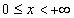and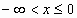.

for which reason it also has two inverse functions: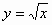and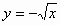.

The defining set of both functions is: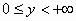and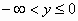Their curves reflects at axis angles 1 and 2 of the quadrant.

If we observe the function:

y = x3,

we see that it is monotone increasing throughout the entire defined set: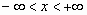Their inverse functions are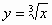for all of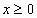, and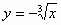for all of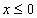Their value set is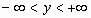The graphs of the parabola curves are created by reflection at the axes of angles 1 and 3 of the quadrant. At the point zero, the function is inversed in the direction of the y axis.

If we consider the functions with roots for every n, which is: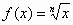,

we find that all of them are inverse functions of the function y =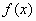= xn, for all n > 0, which means that all are positive.

For even n, the function must be defined in two areas:andand has, as was shown before, the following two inverse functions as a result: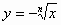and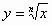The points x = 0 and y = 0 belong to both functions.

For odd n and in the set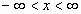, the following inverse functions existforand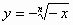for all.

For n > 0, the function is monotone increasing and, for n < 0, monotone decreasing. For n > 0, the point zero lies at x = 0. For n > 0, there are no poles and, for n < 0, it lies at x = 0. The function does not have any extremes and we have only one point of inversion with unlimited ascension while x = 0 and for all odd n > 0. For even n, no symmetry exists and, for odd n, the curve is point symmetric with the centre at the origin of the coordinate system. The derivative of the function is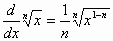.

The inverse of the function is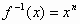.

Translating Dutch Hungarian Translations Hungarian Dutch Translating Swedish Czech Translations Czech Swedish Translating RussianEnter your search terms Submit search form